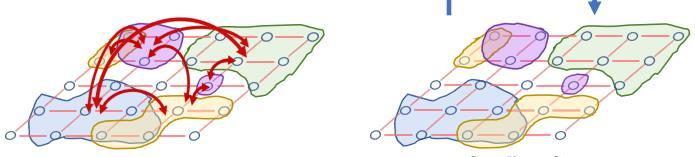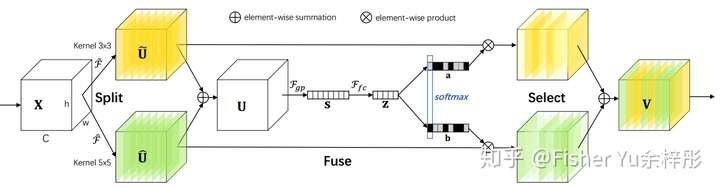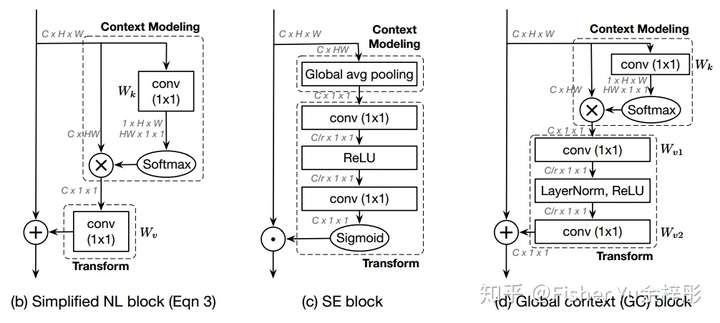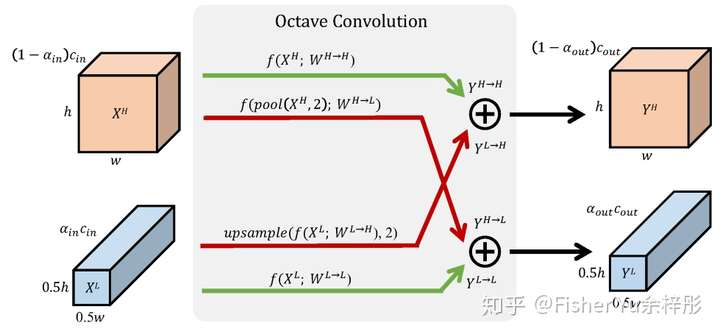# SKNet, GCNet, GloRe, Octave# [Selective Kernel Unit, SKNet]

Xiang Li et al., Selective Kernel Networks, CVPR2019Classical convolution neural network limits the filter of the same layer having the same field of view, which is different from human vision where the reception size of stimulus is adaptive. To address tgus, SK unit is proposed, which contains the following steps:

• split: the input are passed into different branches which has different convolution size;
• fuse: the output of two branches are added and then passed to some fully connected layer to compute a channel weight vector for each of the branches (applying softmax for each channel cross branches);
• select: compute the weighted sum of outputs of two branches according to the weight computed from fuse step.

# [Global Context (GC) block, GCNet]

Yue Cao et al., GCNet: Non-local Networks Meet Squeeze-Excitation Networks and Beyond# [Global Reasoning Unit, GloRe]

Yunpeng Chen et al. ,Graph-Based Global Reasoning Networks, CVPR2019GloRe tries to capture the interaction at global scale, which is difficult to achieve in coordinate space. Thus it proposes to do that in interaction space.

# [Octave Convolution]

Yunpeng Chen et al. ,Drop an Octave: Reducing Spatial Redundancy in Convolutional Neural Networks with Octave ConvolutionOctave convolution tries to reduce the parameter size and computation cost. It achieves this by spliting the convolution into high frequency filter and low frequency filter, where low convolution filter doesn’t need larger kernel size. Experiement result indicates when about 10%~20% are low frequence filters, the performance and computation cost is optimal.

Written on May 16, 2019